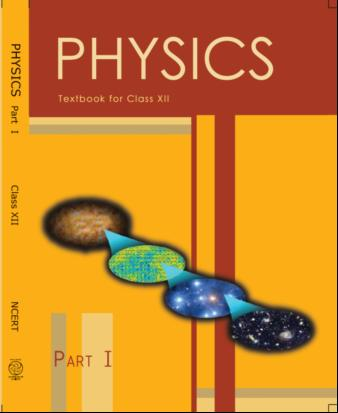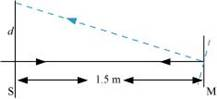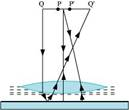# NCERT Solutions class 12 physics Ray Optics and Optical Instruments Part 2## myCBSEguide App

CBSE, NCERT, JEE Main, NEET-UG, NDA, Exam Papers, Question Bank, NCERT Solutions, Exemplars, Revision Notes, Free Videos, MCQ Tests & more.

Download NCERT solutions for Ray Optics and Optical Instruments Part 2 as PDF.## NCERT Class 12 Physics Chapter-wise Solutions

• 1 – Electric Charges and Fields
• 2 – Electrostatic Potential and Capacitance
• 3 – Current Electricity
• 4 – Moving Charges and Magnetism
• 5 – Magnetism and Matter
• 6 – Electromagnetic Induction
• 7 – Alternating Current
• 8 – Electromagnetic Waves
• 9 – Ray Optics and Optical Instruments
• 10 – Wave Optics
• 11 – Dual Nature of Radiation and Matter
• 12 – Atoms
• 13 – Nuclei
• 14 – Semiconductor Electronic: Material, Devices and Simple Circuits
• 15 – Communication Systems

## CHAPTER 9 RAY OPTICS AND OPTICAL INSTRUMENTS

• 9.1 Introduction
• 9.2 Reflection of Light by Spherical Mirrors
• 9.3 Refraction
• 9.4 Total Internal Reflection
• 9.5 Refraction at Spherical Surfaces and by Lenses
• 9.6 Refraction through a Prism
• 9.7 Dispersion by a Prism
• 9.8 Some Natural Phenomena due to Sunlight
• 9.9 Optical Instruments

## NCERT Solutions class 12 physics Ray Optics and Optical Instruments Part 2

20: A screen is placed 90 cm from an object. The image of the object on the screen is formed by a convex lens at two different locations separated by 20 cm. Determine the focal length of the lens.

21:  (a) Determine the ‘effective focal length’ of the combination of the two lenses in Exercise 9.10, if they are placed 8.0 cm apart with their principal axes coincident. Does the answer depend on which side of the combination a beam of parallel light is incident? Is the notion of effective focal length of this system useful at all?

(b) An object 1.5 cm in size is placed on the side of the convex lens in the arrangement (a) above. The distance between the object and the convex lens is 40 cm. Determine the magnification produced by the two-lens system, and the size of the image.

22: At what angle should a ray of light be incident on the face of a prism of refracting angle 60° so that it just suffers total internal reflection at the other face? The refractive index of the material of the prism is 1.524.

23:  You are given prisms made of crown glass and flint glass with a wide variety of angles. Suggest a combination of prisms which will

(a) deviate a pencil of white light without much dispersion,

(b) disperse (and displace) a pencil of white light without much deviation.

24:  For a normal eye, the far point is at infinity and the near point of distinct vision is about 25cm in front of the eye. The cornea of the eye provides a converging power of about 40 dioptres, and the least converging power of the eye-lens behind the cornea is about 20 dioptres. From this rough data estimate the range of accommodation (i.e., the range of converging power of the eye-lens) of a normal eye.

25: Does short-sightedness (myopia) or long-sightedness (hypermetropia) imply necessarily that the eye has partially lost its ability of accommodation? If not, what might cause these defects of vision?

26: A myopic person has been using spectacles of power -1.0 dioptre for distant vision. During old age he also needs to use separate reading glass of power + 2.0 dioptres. Explain what may have happened.

27:  A person looking at a person wearing a shirt with a pattern comprising vertical and horizontal lines is able to see the vertical lines more distinctly than the horizontal ones. What is this defect due to? How is such a defect of vision corrected?

28:  A man with normal near point (25 cm) reads a book with small print using a magnifying glass: a thin convex lens of focal length 5 cm.

(a) What is the closest and the farthest distance at which he should keep the lens from the page so that he can read the book when viewing through the magnifying glass?

(b) What is the maximum and the minimum angular magnification (magnifying power) possible using the above simple microscope?

29:  A card sheet divided into squares each of size 1 mm2is being viewed at a distance of 9 cm through a magnifying glass (a converging lens of focal length 9 cm) held close to the eye.

(a) What is the magnification produced by the lens? How much is the area of each square in the virtual image?

(b) What is the angular magnification (magnifying power) of the lens?

(c) Is the magnification in (a) equal to the magnifying power in (b)?

Explain.

30: (a) At what distance should the lens be held from the figure in

Exercise 9.29 in order to view the squares distinctly with the maximum possible magnifying power?

(b) What is the magnification in this case?

(c) Is the magnification equal to the magnifying power in this case?

Explain.

31: What should be the distance between the object in Exercise 9.30 and the magnifying glass if the virtual image of each square in the figure is to have an area of 6.25 mm2. Would you be able to see the squares distinctly with your eyes very close to the magnifier?

[Note: Exercises 9.29 to 9.31 will help you clearly understand the difference between magnification in absolute size and the angular magnification (or magnifying power) of an instrument.]

(a) The angle subtended at the eye by an object is equal to the angle subtended at the eye by the virtual image produced by a magnifying glass. In what sense then does a magnifying glass provide angular magnification?

(b) In viewing through a magnifying glass, one usually positions one’s eyes very close to the lens. Does angular magnification change if the eye is moved back?

(c) Magnifying power of a simple microscope is inversely proportional to the focal length of the lens. What then stops us from using a convex lens of smaller and smaller focal length and achieving greater and greater magnifying power?

(d) Why must both the objective and the eyepiece of a compound microscope have short focal lengths?

(e) When viewing through a compound microscope, our eyes should be positioned not on the eyepiece but a short distance away from it for best viewing. Why? How much should be that short distance between the eye and eyepiece?

33:  An angular magnification (magnifying power) of 30X is desired using an objective of focal length 1.25 cm and an eyepiece of focal length 5 cm. How will you set up the compound microscope?

34:  An angular magnification (magnifying power) of 30X is desired using an objective of focal length 1.25 cm and an eyepiece of focal length 5 cm. How will you set up the compound microscope?

35:  A small telescope has an objective lens of focal length 140 cm and an eyepiece of focal length 5.0 cm. What is the magnifying power of the telescope for viewing distant objects when

(a) the telescope is in normal adjustment (i.e., when the final image is at infinity)?

(b) the final image is formed at the least distance of distinct vision (25 cm)?

36: (a) For the telescope described in Exercise 9.34 (a), what is the separation between the objective lens and the eyepiece?

(b) If this telescope is used to view a 100 m tall tower 3 km away, what is the height of the image of the tower formed by the objective lens?

(c) What is the height of the final image of the tower if it is formed at 25 cm?

37:  A Cassegrain telescope uses two mirrors as shown in Fig. 9.33. Such a telescope is built with the mirrors 20 mm apart. If the radius of curvature of the large mirror is 220 mm and the small mirror is 140 mm, where will the final image of an object at infinity be?

38: Light incident normally on a plane mirror attached to a galvanometer coil retraces backwards as shown in Fig. 9.36. A current in the coil produces a deflection of 3.5°of the mirror. What is the displacement of the reflected spot of light on a screen placed 1.5 m away?39:  Figure 9.37 shows an equiconvex lens (of refractive index 1.50) in contact with a liquid layer on top of a plane mirror. A small needle with its tip on the principal axis is moved along the axis until its inverted image is found at the position of the needle. The distance of the needle from the lens is measured to be 45.0 cm. The liquid is removed and the experiment is repeated. The new distance is measured to be 30.0 cm. What is the refractive index of the liquid?## NCERT Solutions for Class 12 Physics

NCERT Solutions Class 12 Physics PDF (Download) Free from myCBSEguide app and myCBSEguide website. Ncert solution class 12 physics includes text book solutions from both part 1 and part 2. NCERT Solutions for CBSE Class 12 Physics have total 20 chapters. 12 Physics NCERT Solutions in PDF for free Download on our website. Ncert physics class 12 solutions PDF and physics ncert class 12 PDF solutions with latest modifications and as per the latest CBSE syllabus are only available in myCBSEguide

To download NCERT Solutions for class 12 Physics, Chemistry, Biology, History, Political Science, Economics, Geography, Computer Science, Home Science, Accountancy, Business Studies and Home Science; do check myCBSEguide app or website. myCBSEguide provides sample papers with solution, test papers for chapter-wise practice, NCERT solutions, NCERT Exemplar solutions, quick revision notes for ready reference, CBSE guess papers and CBSE important question papers. Sample Paper all are made available through the best app for CBSE students and myCBSEguide website.### 1 thought on “NCERT Solutions class 12 physics Ray Optics and Optical Instruments Part 2”

1. In what way does the magnification produced by a compound microscope depend on the tube length?